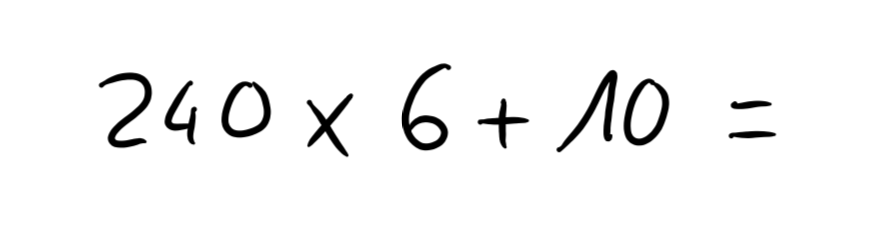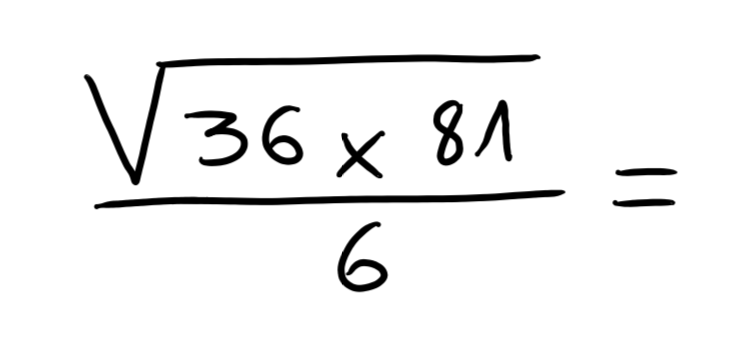# Math

 Need to write a calculation or equation? No problem!To add a math object to a Nebo Document, tap + in the upper right corner of your page and select Math. Alternatively, double-tap with your finger in an empty space to open a contextual menu, then select Math.Nebo can solve simple calculations for you. Double-tap to convert calculations and display the results automatically. Try it on the calculation below:Tap your math object to select it, then tap … in the upper right corner of the object to display a contextual menu. This menu lets you choose how results are presented (truncated or rounded), the units in which angles are measured (degrees or radians) and whether calculations should be automatically solved or not.To erase, simply use the erase gesture.You can also write complex equations like this one: